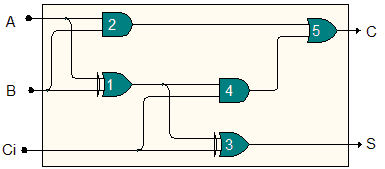Proposed by Francisco Azevedo

When the output of some system does not correspond to its expected behaviour for a given input, one is faced with the problem of diagnosis. We are particularly concerned with digital circuits where only the circuits input and output may be observed.

Given a set of possible faults, we want to differentiate them in order to find the real one affecting the circuit. For that, we may perform pairwise differentiations between 2 diagnoses (2 sets of faults - usually 2 single faults) d1,d2, by generating an input vector Id1,d2 that would entail an output vector Od1 under diagnosis d1 (i.e. with faults d1 really present in the circuit), and an output vector Od2 under diagnosis d2, with Od1 different than Od2. Applying such input Id1,d2 to the circuit, at least one of the 2 diagnoses will be discarded by checking the output.

Differential diagnosis thus consists in finding such a differentiating test vector, or concluding that none exists (in which case we say that the two diagnoses are indistinguishable). (As usual, faulty circuit gates are either stuck-at-0 _or _stuck-at-1, respectively outputting value 0 or 1 independently of the input.)

In the example adder circuit below, to differentiate candidate faults Gate1 stuck-at-1 and Gate3 stuck-at-1, a possible input test vector is , since with Gate1 stuck-at-1 the output would be $(S=0,C=1)$, whereas with _Gate3 stuck-at-1 _the output would be (the same as without faults).